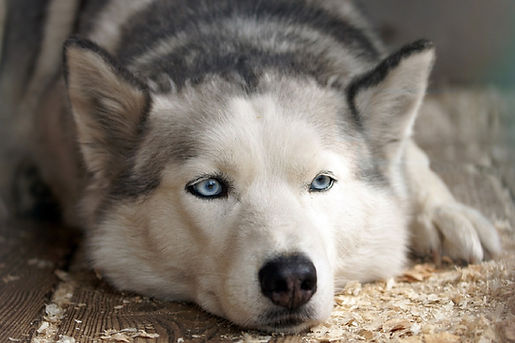# Our MemorialGeisha

BORN:

January, 2006

DIED:

October 17, 2018

This is the place where you can tell us about the pet. This is the place where you can tell us about the pet.  This is the place where you can tell us about the pet. This is the place where you can tell us about the pet.  This is the place where you can tell us about the pet.  This is the place where you can tell us about the pet.  This is the place where you can tell us about the pet.  This is the place where you can tell us about the pet.  This is the place where you can tell us about the pet.  This is the place where you can tell us about the pet.  This is the place where you can tell us about the pet.   This is the place where you can tell us about the pet. This is the place where you can tell us about the pet.  This is the place where you can tell us about the pet.FIDO FIDO

BORN:

December 4, 2019

DIED:

December 4, 2019

This is the place where you can tell us about the pet. This is the place where you can tell us about the pet.  This is the place where you can tell us about the pet. This is the place where you can tell us about the pet.  This is the place where you can tell us about the pet.  This is the place where you can tell us about the pet.  This is the place where you can tell us about the pet.  This is the place where you can tell us about the pet.  This is the place where you can tell us about the pet.  This is the place where you can tell us about the pet.  This is the place where you can tell us about the pet.   This is the place where you can tell us about the pet. This is the place where you can tell us about the pet.  This is the place where you can tell us about the pet.